International
Tables for
Crystallography
Volume B
Reciprocal space
Edited by U. Shmueli

International Tables for Crystallography (2010). Vol. B, ch. 1.3, p. 54   | 1 | 2 |

## Section 1.3.3.2.2.2. The Chinese remainder theorem

G. Bricognea

aGlobal Phasing Ltd, Sheraton House, Suites 14–16, Castle Park, Cambridge CB3 0AX, England, and LURE, Bâtiment 209D, Université Paris-Sud, 91405 Orsay, France

#### 1.3.3.2.2.2. The Chinese remainder theorem

| top | pdf |

Letbe factored into a product of pairwise coprime integers, so that g.c.d.for. Then the system of congruence equationshas a unique solutionmod N. In other words, eachis associated in a one-to-one fashion to the d-tupleof its residue classes in.

The proof of the CRT goes as follows. Let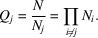Since g.c.d.there exist integersandsuch thatthen the integer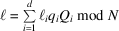is the solution. Indeed,because all terms withcontainas a factor; andby the defining relation for.

It may be noted that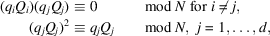so that theare mutually orthogonal idempotents in the ring, with properties formally similar to those of mutually orthogonal projectors onto subspaces in linear algebra. The analogy is exact, since by virtue of the CRT the ringmay be considered as the direct productvia the two mutually inverse mappings:

 (i)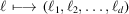bymodfor each j; (ii)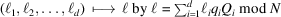.

The mapping defined by (ii)is sometimes called the `CRT reconstruction' offrom the.

These two mappings have the property of sending sums to sums and products to products, i.e: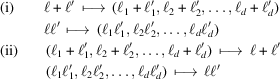(the last proof requires using the properties of the idempotents). This may be described formally by stating that the CRT establishes a ring isomorphism: